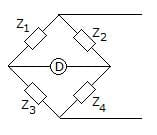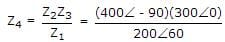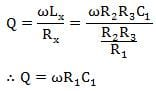GATE  >  Test: AC Bridges

# Test: AC Bridges

Test Description

## 25 Questions MCQ Test Sensor & Industrial Instrumentation | Test: AC Bridges

Test: AC Bridges for GATE 2022 is part of Sensor & Industrial Instrumentation preparation. The Test: AC Bridges questions and answers have been prepared according to the GATE exam syllabus.The Test: AC Bridges MCQs are made for GATE 2022 Exam. Find important definitions, questions, notes, meanings, examples, exercises, MCQs and online tests for Test: AC Bridges below.
Solutions of Test: AC Bridges questions in English are available as part of our Sensor & Industrial Instrumentation for GATE & Test: AC Bridges solutions in Hindi for Sensor & Industrial Instrumentation course. Download more important topics, notes, lectures and mock test series for GATE Exam by signing up for free. Attempt Test: AC Bridges | 25 questions in 30 minutes | Mock test for GATE preparation | Free important questions MCQ to study Sensor & Industrial Instrumentation for GATE Exam | Download free PDF with solutions
 1 Crore+ students have signed up on EduRev. Have you?
Test: AC Bridges - Question 1

### In figure, Z1 = 200∠60° Ω, Z2 = 400∠ - 90° Ω, Z3 = 300∠0°. Then Z4 for the bridge to be balanced isDetailed Solution for Test: AC Bridges - Question 1or Z4 = 600∠-150° ohm

Test: AC Bridges - Question 2

### Frequency can be measured using

Detailed Solution for Test: AC Bridges - Question 2 Frequency is usually represented as angular frequency ω in radians/second or as ƒ in seconds-1, also known as the unit hertz (Hz). You also can use beats per minute (BPM) and revolutions per minute (RPM) to represent frequency. Angular frequency ω (rad/sec) and ƒ (Hz) are related by the following formula: ω =2πƒ.
Test: AC Bridges - Question 3

### Schering bridge can be used for measurement of

Detailed Solution for Test: AC Bridges - Question 3 The Schering bridge use for measuring the capacitance of the capacitor, dissipation factor, properties of an insulator, capacitor bushing, insulating oil, and other insulating materials. It is one of the most commonly used AC bridges. The Schering bridge works on balancing the load on its arm.
Test: AC Bridges - Question 4

The main advantage of Owen’s bridge for measurement of unknown inductance is that

Detailed Solution for Test: AC Bridges - Question 4 The advantages of the Owens bridge are, the unknown inductance measured is independent of frequency and doesn't require any frequency supply. The balance equation can be obtained very quickly and simply. It is used to measure a wide range of inductance in capacitance.
Test: AC Bridges - Question 5

The advantage of Hay’s bridge over Maxwell’s inductance–capacitance bridge is that

Detailed Solution for Test: AC Bridges - Question 5 The bridge gives a very simple expression for calculating unknown inductors of high value. The Hay's bridge requires a low value of r4, while the Maxwell bridge requires a high value of r4.
Test: AC Bridges - Question 6

De Sauty’s bridge is used for the measurement of

Detailed Solution for Test: AC Bridges - Question 6 This bridge provides us the most suitable method for comparing the two values of the capacitor if we neglect dielectric losses in the bridge circuit.
Test: AC Bridges - Question 7

The advantage of Anderson’s bridge over Maxwell’s bridge is that

Detailed Solution for Test: AC Bridges - Question 7 By Anderson's bridge, one can measure inductance by utilizing other circuit components like resistors and capacitors. In Maxwell's bridge, the inductance is calculated by comparing it with a standard variable capacitance.
Test: AC Bridges - Question 8

Maxwell’s bridge can be used for measurement of inductance with

Detailed Solution for Test: AC Bridges - Question 8 A Maxwell Inductance Capacitance Bridge (known as a Maxwell Bridge) is a modified version of a Wheatstone bridge that is used to measure the self-inductance of a circuit. A Maxwell bridge uses the null deflection method (also known as the “bridge method”) to calculate an unknown inductance in a circuit.
Test: AC Bridges - Question 9

Under balanced condition of a bridge for measuring unknown impedance, if the detector is suddenly taken out

Detailed Solution for Test: AC Bridges - Question 9 The zero response of the detector indicates balance. At balance, no current flows through the detector, i.e., there is no potential difference across the detector, or in other words, the potentials at points B and C are the same.
Test: AC Bridges - Question 10

Frequency can be measured by using:

Detailed Solution for Test: AC Bridges - Question 10 The Wien bridge is one of many standard bridges. Wien's bridge is used for precision measurement of capacitance in resistance and frequency. It was also used to measure audio frequencies.
Test: AC Bridges - Question 11

Maxwell’s inductance-capacitance bridge is used for measurement of inductance of :

Detailed Solution for Test: AC Bridges - Question 11 A Maxwell bridge is a modification to a Wheatstone bridge used to measure an unknown inductance (usually of low Q value) in terms of calibrated resistance and inductance or resistance and capacitance. When the calibrated components are a parallel resistor and capacitor, the bridge is known as a Maxwell-Wien bridge.
Test: AC Bridges - Question 12

The advantage of Hay’s bridge over Maxwell’s inductance-capacitance bridge is because

Detailed Solution for Test: AC Bridges - Question 12 The bridge gives very simple expression for the calculation of unknown inductors of high value. The Hay's bridge requires a low value of r4 while the Maxwell bridge requires a high value of r4.
Test: AC Bridges - Question 13

In D’Sauty’s bridge (unmodified form), it is :

Detailed Solution for Test: AC Bridges - Question 13 De Sauty Bridge is a very simple type of AC Bridge used to measure capacitance. Here we measure unknown capacitance in terms of known capacitance and known resistance. Hence, we design a De Sauty Bridge by using two known resistances (R1 and R4), one known capacitance (C2), and one unknown capacitance (C3).
Test: AC Bridges - Question 14

Wagner’s Earth devices are used in a.c bridge circuit for :

Detailed Solution for Test: AC Bridges - Question 14 The Wagner earthing device is used for removing the earth capacitance from the bridges. It is a type of voltage divider circuit used to reduce the error resulting from stray capacitance. The Wagner Earth device provides high accuracy to the bridge.
Test: AC Bridges - Question 15

Quality factor of the circuit is given by _________

Detailed Solution for Test: AC Bridges - Question 15 The relation gives the quality factor of a Maxwell inductance capacitance bridgeTest: AC Bridges - Question 16

At high frequency, source consists of ________.

Detailed Solution for Test: AC Bridges - Question 16 Op-amps are differential amplifiers. Amplifiers are used in analog circuits for increasing the strength of the signal. Electronic oscillators form sources at high frequencies.
Test: AC Bridges - Question 17

In AC bridges, the Wagner earth devices are used to

Detailed Solution for Test: AC Bridges - Question 17 The Wagner earthing device is used for removing the earth capacitance from the bridges. It is a type of voltage divider circuit used to reduce the error resulting from stray capacitance. The Wagner Earth device provides high accuracy to the bridge.
Test: AC Bridges - Question 18

Tuned detectors are used in the frequency range of ________.

Detailed Solution for Test: AC Bridges - Question 18 Tuned amplifier circuits are used as detectors in the low-frequency range. The low-frequency range usually ranges from 10 Hz to 100 Hz in AC bridges.
Test: AC Bridges - Question 19

AC bridge is an outcome of ________.

Detailed Solution for Test: AC Bridges - Question 19 Wheatstone bridge is the simplest form of the bridge for measuring resistance and forms the basis for an AC bridge. Kelvin bridge is used to measure low resistance, and a megger is used for the measurement of high resistances.
Test: AC Bridges - Question 20

What is the frequency range for a headphone as a detector?

Detailed Solution for Test: AC Bridges - Question 20 Headphones can be used as detectors in AC bridges in the low audio frequency range. The common audio frequency range varies from 250 Hz to 4 kHz.
Test: AC Bridges - Question 21

The Wien’s bridges are suitable for the measurement of the frequency of the range of

Detailed Solution for Test: AC Bridges - Question 21 Wien's bridge is suitable for measuring frequencies from 100 Hz to 100 kHz. It is possible to obtain an accuracy of 0.1 to 0.5 percent. Because of its frequency sensitivity, the Wien's bridge may be challenging to balance unless the applied voltage waveform is sinusoidal.
Test: AC Bridges - Question 22

For single frequency value, the most sensitive detector is ________.

Detailed Solution for Test: AC Bridges - Question 22 A vibration galvanometer is used for detecting the balance condition. The oscillator is used as a source of the supply voltage. The tuned detector is the most sensitive detector for a single frequency value.
Test: AC Bridges - Question 23

Source is ________.

Detailed Solution for Test: AC Bridges - Question 23 For an AC bridge, we require an AC supply as the voltage source. It supplies AC voltage at the required frequency.
Test: AC Bridges - Question 24

In the simplest form, an AC bridge consists of ____________.

Detailed Solution for Test: AC Bridges - Question 24 In its simplest form, an AC bridge consists of four arms, a source for excitation, and a null detector. The source is connected across a pair of arms while the detector is connected to the team of opposite arms.
Test: AC Bridges - Question 25

Commonly used balance detectors for AC bridges are headphones, tuned amplifiers, and vibration galvanometers.

Detailed Solution for Test: AC Bridges - Question 25 Headphones, tuned amplifier circuits and vibration galvanometers are used for detecting the balance condition in AC bridges.

## Sensor & Industrial Instrumentation

23 videos|24 docs|29 tests
 Use Code STAYHOME200 and get INR 200 additional OFF Use Coupon Code
Information about Test: AC Bridges Page
In this test you can find the Exam questions for Test: AC Bridges solved & explained in the simplest way possible. Besides giving Questions and answers for Test: AC Bridges, EduRev gives you an ample number of Online tests for practice

## Sensor & Industrial Instrumentation

23 videos|24 docs|29 tests